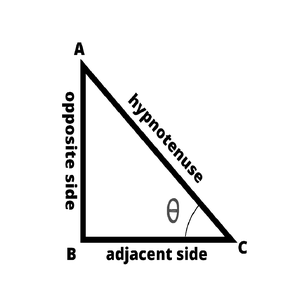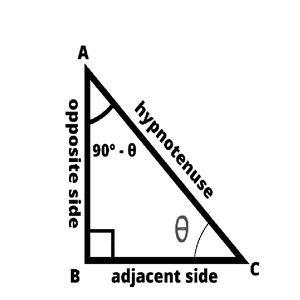GFG App
Open AppBrowser
Continue

# Find the value of tan 31pi/3

Trigonometry is a field in mathematics that deals with the ratios of the sides and their angles in a triangle. By using various relations and identities in a triangle of its sides and its corresponding angles, many problems(distance, height, etc) can be solved and calculated much more precisely and easily. There are several standard ratios or relations between the angles and the sides of the triangle that help to solve some basic as well as complex problems.

### Trigonometric Ratios

A Trigonometric ratio is defined as the proportion of the sides to the angles of a right-angled triangle. A standard trigonometric ratio can be obtained as the ratio of the sides to either of the acute angles of the right-angled triangles. Some definitions of some standard trigonometric ratios such as sine, cosine, and tangent as follows,• Sine is the function that takes in the parameter an angle θ, which is either of the acute-angles in the right-angled triangles and is defined as the ratio of the length of the opposite side to the hypotenuse of the right-angled triangle. In technical terms, it can be written as follows,

sin(θ) = opposite side / hypotenuse

• Cosine is the function that takes in the parameter an angle θ, which is either of the acute-angles in the right-angled triangles and is defined as the ratio of the length of the adjacent side to the hypotenuse of the right-angled triangle. In technical terms, it can be written as follow,

cos(θ) = adjacent side / hypotenuse

• Tangent is the function that takes in the parameter an angle θ, which is either of the acute-angles in the right-angled triangles and is defined as the ratio of the length of the opposite side to the adjacent side of the right-angled triangle. In technical terms, it can be written as follows,

tan(θ) = opposite side / adjacent side

These trigonometric ratios are related to one another using some trigonometric identities and formulas,

tan(θ) = sin(θ) / cos(θ)

sin2(θ) + cos2(θ) = 1

Each of the trigonometric ratios has other three derived trigonometric ratios which are deduced by taking the inverse of the respective ratios. The Other three Trigonometric ratios are Cosecant, Secant, and Cotangent used mathematically as cosec, sec, and cot. These are related to the Primary trigonometric ratios as follows,

cosec(θ) = 1 / sin(θ)

sec(θ) = 1 / cos(θ)

cot(θ) = 1 / tan(θ) = cos(θ) / sin(θ)

Below are some of the identities related to the standard trigonometric ratios and the derived trigonometric ratios,

tan2(θ) + 1 = sec2(θ)

cot2(θ) + 1 = cosec2(θ)

### Trigonometric Table

The below is the table for some common angles and the basic trigonometric ratios. The value of each angle in the trigonometry is fixed and known but the ones mentioned are more common and mostly used,

There are also some other trigonometric ratios to apply beyond the right-angled triangles:

sin(-θ) = – sin(θ)

cos(-θ) = cos(θ)

tan(-θ) = – tan(θ)

### Complementary and Supplementary angles

Certain formulas are defined for complementary and supplementary angles. The complementary angles are the angles that add up to form 90° or π/2 radians. We can form such angles and find the equivalent angles in terms of the trigonometric ratios.

The supplementary angles are the angles that add up to form 180° or π radians. We can form such angles and find the equivalent angles in terms of the trigonometric ratios.Subtract an appropriate angle from 90° (π/2 radians) or add an angle to 180° (π radians) to obtain the complementary angles. Add an appropriate angle to 90° (π/2 radians) or subtract an angle from 180° (π radians) to obtain the pair of supplementary angles. The actual angle can be adjusted in the function of trigonometric ratios to form either complementary or supplementary angles and then evaluate the deduced trigonometric ratio as per the list of formulas given below. There are some trigonometric ratios for complementary and supplementary angles,

• sin(nπ/2 + θ) = cos(θ) or sin(n90° + θ) = cos(θ)
• sin(nπ/2 – θ) = cos(θ) or sin(n × 90° – θ) = cos(θ)
• cos(nπ/2 + θ) = -sin(θ) or cos(n × 90°+ θ) = -sin(θ)
• cos(nπ/2 – θ) = sin(θ) or cos(n × 90° – θ) = sin(θ)
• tan(nπ/2 + θ) = -cot(θ) or tan(n × 90° + θ) = -cot(θ)
• tan(nπ/2 – θ) = cot(θ) or tan(n × 90° – θ) = cot(θ)
• sin(nπ + θ) = -sin(θ) or sin(n × 180° + θ) = -sin(θ)
• sin(nπ – θ) = sin(θ) or sin(n × 180° – θ) = sin(θ)
• cos(nπ + θ) = -cos(θ) or cos(n × 180° + θ) = -cos(θ)
• cos(nπ – θ) = -cos(θ) or cos(n × 180° – θ) = -cos(θ)
• tan(nπ + θ) = tan(θ) or tan(n × 180° + θ) = tan(θ)
• tan(nπ – θ) = -tan(θ) or sin(n × 180° – θ) = -tan(θ)
• sin(3nπ/2 + θ) = -cos(θ) or sin(n × 270° + θ) = -cos(θ)
• sin(3nπ/2 – θ) = -cos(θ) or sin(n × 270° – θ) = -cos(θ)
• cos(3nπ/2 + θ) = sin(θ) or cos(n × 270° + θ) = sin(θ)
• cos(3nπ/2 – θ) = -sin(θ) or cos(n × 270° – θ) = -sin(θ)
• tan(3nπ/2 – θ) = cot(θ) or tan(n × 270° + θ) = cot(θ)
• tan(3nπ/2 + θ) = -cot(θ) or tan(n × 270° – θ) = -cot(θ)
• sin(2nπ + θ) = sin(θ) or sin(n × 360° + θ) = sin(θ)
• sin(2nπ – θ) = -sin(θ) or sin(n × 360° – θ) = -sin(θ)
• cos(2nπ + θ) = cos(θ) or cos(n × 360°+ θ) = cos(θ)
• cos(2nπ – θ) = cos(θ) or cos(n × 360° – θ) = cos(θ)
• tan(2nπ + θ) = tan(θ) or tan(n × 360°+ θ) = tan(θ)
• tan(2nπ – θ) = -tan(θ) or tan(n × 360° – θ) = -tan(θ)

Some more important formulae,

1. tan(A + B) = [tan(A) + tan(B)] / [1 – (tan(A)tan(B))]
2. tan(A – B) = [tan(A) – tan(B)] / [1 + (tan(A)tan(B))]

### Find the value of tan 31π/3

Method 1

tan(31π/3)

It can be written 31π/3 as (10π + π/3),

Thus,

tan(31π/3) = tan(10π + π/3)

= tan [ 5(2π) + π/3 ]

tan(2nπ + θ) = tan(θ)

Thus,

tan(31π/3) = tan [ 5(2π) + π/3 ]

= tan(π/3)

= √3

Therefore,

tan(31π/3) = √3

Method 2

tan(31π/3)

It can be written, 31π/3 as (21π/2 – π/6),

Thus,

tan(31π/3) = tan [ 21π/2 – π/6 ]

= tan [ 7(3π/2) – π/6 ]

tan(3nπ/2 – θ ) = cot ( θ )

Thus,

tan(31π/2) = tan [ 7(3π/2) – π/6 ]

= cot ( π/6 )

= √3

Therefore,

tan(31π/3) = √3

Method 3

31π/3 can be written as 14π/3 + 17π/3,

Now,

14π/3 = 9π/2 + π/6 and,

17π/3 = 11π/2 + π/6

Therefore,

tan(31π/3) = tan [ (9π/2 + π/6 ) + (11π/2 + π/6) ]

tan(A + B) = [tan(A) + tan(B)] / [1 – (tan(A).tan(B))]

Here, A= 9π/2 + π/6 and B = 11π/2 + π/6,

Thus, tan(A) = tan(9π/2 + π/6 ) = tan(3(3π/2) + π/6 )

tan(B) = tan(11π/2 + π/6) = tan(11(π/2) + π/6 )

tan(3nπ/2 + θ) = -cot(θ)

tan(nπ/2 + θ) = -cot(θ)

Therefore,

tan(A) = tan((9π/2 + π/6) = -cot(π/6) = -√3

tan(B) = tan((11π/2 + π/6)= -cot(π/6) = -√3

Now,

tan(31π/3) = tan [ (9π/2 + π/6 ) + (11π/2 + π/6) ]

= [ tan(9π/2 + π/6 ) + tan(11π/2 + π/6) ] / [ 1 – (tan((9π/2 + π/6).tan(11π/2 + π/6) ]

= [ (-√3) + (-√3) ] / [ 1 – (-√3).(-√3) ]

= [ (-2√3 ] / [ 1 – 3 ]

= [ -2√3 ] / [ -2 ]

= √3

Therefore,

tan(31π/3) = √3

Thus the value of tan(31π/3) to be √3 which is almost 1.732…

### Similar Problems

Question 1: Find the value of  tan(5π/4)

Solution:

5π/4 can be written as π + π/4

Thus, tan(5π/4) = tan(π + π/4)

tan(π + θ) = tan(θ) Thus,

tan(5π/4) = tan( π + π/4)

= tan( π/4)

=1

tan(5π/4) = 1

Question 2: Find the value of tan(π/12)

Solution:

π/12 can be written as π/4 – π/6,

Thus, tan(π/12) = tan (π/4 – π/6)

tan(A + B) = [tan(A) + tan(B)] / [1 – (tan(A).tan(B))]

Here,

A = π/4 and B = π/6 Thus,

tan(π/12) = tan (π/4 – π/6)

= [tan (π/4) – tan(π/6)] / [1 – (tan(π/4).tan(π/6))]

= [1 – 1/√3] / [1 – ((1).(1/√3))]

= [√3 -1] / [(√3 -1) / √3]

= (√3 -1) √3 / (√3 – 1)

= (3 -√3) / (√3 -1)

Therefore,

tan(π/12) =  (3 – √3) / (√3 – 1)

Question 3: Find tan(300°)

Solution:

We can write 300° = 270° + 30°

Note Here, angles are used in degree and not in radians

tan(300°) = tan(270° + 30°)

tan(270° +  θ) = -cot(θ) Thus,

tan(300°) = tan(270° + 30°)

= -cot(30°)

= -√3

Thus,

tan(300°) = -√3

My Personal Notes arrow_drop_up